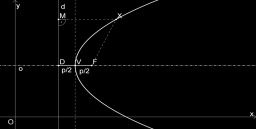# At what

At what speed did the motorist drive when he reacted by noticing the obstacle by 0.8 s; the magnitude of the opposite acceleration during braking was 6.5 m/s2, and the car ran to a stopped track 35 m?

v =  16.7554 m/s

### Step-by-step explanation:Did you find an error or inaccuracy? Feel free to write us. Thank you!

Tips for related online calculators
Are you looking for help with calculating roots of a quadratic equation?
Do you have a linear equation or system of equations and looking for its solution? Or do you have a quadratic equation?
Do you want to convert time units like minutes to seconds?